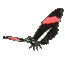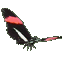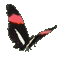Teaching Primary Trigonometry Functions - Sine Cosine Tangent Help - Game Tips:

- When angle A is in standard position at the graph's origin (0,0) then sin A equals (y/r).
Since r is the hypotenuse of a right triangle, r can be found from point D(x,y) using x2 + y2 = r2 .
Consequently, sin A can also be described as (opposite side)/(hypotenuse).
sin(A) = o/h = y/r .

- When angle A is in standard position at the graph's origin (0,0) then cos A equals (x/r).
Also cos A can be described as (adjacent side)/(hypotenuse).
cos(A) = a/h = x/r .

- When angle A is in standard position at the graph's origin (0,0) then tan A equals the ratio = (y/x).
tan(A) = o/a = y/x .

- A memory aid for Sine, Cosine & Tangent ratios in a right triangle is ' soh,cah,toa '.

- Your Game Score is reduced by the number of butterfly hits.

- To slow the game speed repeat tap/click on the word Slider.
- To increase the game speed repeat tap/click on the word Math.
- Speed can also be adjusted with a keyboard's - and + keys.

- Refresh/Reload the web page to restart the game.